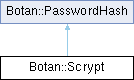Botan 2.19.1 Crypto and TLS for C&
Botan::Scrypt Class Referencefinal

`#include <scrypt.h>`

Inheritance diagram for Botan::Scrypt:## Public Member Functions

void derive_key (uint8_t out[], size_t out_len, const char *password, size_t password_len, const uint8_t salt[], size_t salt_len) const override

size_t iterations () const override

size_t memory_param () const override

size_t N () const

Scryptoperator= (const Scrypt &)=default

size_t p () const

size_t parallelism () const override

size_t r () const

Scrypt (const Scrypt &other)=default

Scrypt (size_t N, size_t r, size_t p)

std::string to_string () const override

size_t total_memory_usage () const override

## Detailed Description

Scrypt key derivation function (RFC 7914)

Definition at line 20 of file scrypt.h.

## ◆ Scrypt() [1/2]

 Botan::Scrypt::Scrypt ( size_t N, size_t r, size_t p )

Definition at line 127 of file scrypt.cpp.

127 :
128 m_N(N), m_r(r), m_p(p)
129 {
130 if(!is_power_of_2(N))
131 throw Invalid_Argument("Scrypt N parameter must be a power of 2");
132
133 if(p == 0 || p > 1024)
134 throw Invalid_Argument("Invalid or unsupported scrypt p");
135 if(r == 0 || r > 256)
136 throw Invalid_Argument("Invalid or unsupported scrypt r");
137 if(N < 1 || N > 4194304)
138 throw Invalid_Argument("Invalid or unsupported scrypt N");
139 }
size_t N() const
Definition: scrypt.h:37
size_t r() const
Definition: scrypt.h:38
size_t p() const
Definition: scrypt.h:39
constexpr bool is_power_of_2(T arg)
Definition: bit_ops.h:43

## ◆ Scrypt() [2/2]

 Botan::Scrypt::Scrypt ( const Scrypt & other )
default

## ◆ derive_key()

 void Botan::Scrypt::derive_key ( uint8_t out[], size_t out_len, const char * password, size_t password_len, const uint8_t salt[], size_t salt_len ) const
overridevirtual

Derive a new key under the current Scrypt parameter set

Definition at line 153 of file scrypt.cpp.

156 {
157 scrypt(output, output_len,
159 salt, salt_len,
160 N(), r(), p());
161 }
void scrypt(uint8_t output[], size_t output_len, const char *password, size_t password_len, const uint8_t salt[], size_t salt_len, size_t N, size_t r, size_t p)
Definition: scrypt.cpp:211
size_t salt_len
Definition: x509_obj.cpp:25

References N(), p(), r(), salt_len, and Botan::scrypt().

## ◆ iterations()

 size_t Botan::Scrypt::iterations ( ) const
inlineoverridevirtual

Most password hashes have some notion of iterations.

Definition at line 41 of file scrypt.h.

41{ return r(); }

## ◆ memory_param()

 size_t Botan::Scrypt::memory_param ( ) const
inlineoverridevirtual

Some password hashing algorithms have a parameter which controls how much memory is used. If not supported by some algorithm, returns 0.

Definition at line 45 of file scrypt.h.

45{ return N(); }

## ◆ N()

 size_t Botan::Scrypt::N ( ) const
inline

Definition at line 37 of file scrypt.h.

37{ return m_N; }

Referenced by derive_key().

## ◆ operator=()

 Scrypt & Botan::Scrypt::operator= ( const Scrypt & )
default

## ◆ p()

 size_t Botan::Scrypt::p ( ) const
inline

Definition at line 39 of file scrypt.h.

39{ return m_p; }

Referenced by derive_key().

## ◆ parallelism()

 size_t Botan::Scrypt::parallelism ( ) const
inlineoverridevirtual

Some password hashing algorithms have a parallelism parameter. If the algorithm does not support this notion, then the function returns zero. This allows distinguishing between a password hash which just does not support parallel operation, vs one that does support parallel operation but which has been configured to use a single lane.

Definition at line 43 of file scrypt.h.

43{ return p(); }

## ◆ r()

 size_t Botan::Scrypt::r ( ) const
inline

Definition at line 38 of file scrypt.h.

38{ return m_r; }

Referenced by derive_key().

## ◆ to_string()

 std::string Botan::Scrypt::to_string ( ) const
overridevirtual

Definition at line 141 of file scrypt.cpp.

142 {
143 std::ostringstream oss;
144 oss << "Scrypt(" << m_N << "," << m_r << "," << m_p << ")";
145 return oss.str();
146 }

## ◆ total_memory_usage()

 size_t Botan::Scrypt::total_memory_usage ( ) const
overridevirtual

Returns an estimate of the total memory usage required to perform this key derivation.

If this algorithm uses a small and constant amount of memory, with no effort made towards being memory hard, this function returns 0.